×

Search anything:

# Remove K digits to make smallest number

#### Algorithms Monotonic Stack Stack Greedy AlgorithmsIn this article, we will be solving the problem of removing K digits from a given number to form the smallest number possible without changing the order of the original number. We will use the idea of Monotonic Stack.

Table of content:

1. Understanding the problem
2. Algorithm & Pseudocode
3. Implementations
4. Explanation / Code walk-through
5. Time and Space Complexity

# Understanding the problem

In this problem, the user provides us with two input numbers NUM and K, and we have to form the smallest number possible from the input number NUM by removing K digits from it without changing the order of the original number.

Suppose the user has provided us with the number NUM = 9154 and K = 2.

What exactly is K here? K is the number of times that we are allowed to remove a digit from the given number in order for us to find the smallest number. So in the number 9154, we first remove the digit 9, which is a peak digit.
What exactly is a peak digit?

Any digit in the number that is greater than the digit present ahead of it in the number is a peak digit. We have to remove as many peak digits from the number as we can to find the smallest number.

After removing 9, K becomes 1 and the number becomes 154. After that, the digit 5 is removed since it is a peak digit, K becomes 0 and the number becomes 14.
Since K has become 0, we cannot remove any more digits from the number, and hence the resultant number that we have is our final answer, which in this case, is 14.
You might be wondering as to what will happen if the input number provided by the user is something like 11111, where we cannot locate a peak digit. What do we do in such cases?

Well, all the corner cases will be handled as well in this article, so keep on reading!

This problem can be solved in many ways, however, we will be solving it by making use of a greedy algorithm along with the stack data structure.

# Algorithm & Pseudocode

In short, we use the idea of Monotonic Stack to solve this problem in linear time.

The idea is to insert each digit into a Stack provided the top of Stack is greater than current element. If top of stack is less than current element, then pop the top of stack and insert current element on checking the condition again. Note the pop operation can be done only K times as per the problem (removing K digits).

The intuitive form of the algorithm is as follows:-

1. Convert the input number into a type where each digit of the number can be iterated properly
2. Iterate through each digit of the input number
2.1 If the digit is lesser than the digit present at the top of the stack, delete the element at the top of the stack and keep track of K
2.2 Add the digit of the input number to the stack
3. Perform a check for corner cases
4. Return the stack in the form of an integer as our final answer

A more elaborate form of the Algorithm / Pseudocode is as follows:-

``````def smallestNumber(num, K) as:
1. If K == 0 do:
1.1 return num
2. If K == length of num do:
2.1 return 0
3. Convert num into a type so that it will be easier to traverse through each digit
4. Declare an empty stack with an appropriate data type, if required
5. For each digit in num do:
5.1 While K != 0 and length of stack > 0 and top element of stack > digit do:
5.1.2 Decrement K by 1 and remove the top element from the stack
5.2 Add digit to the stack
6. If K > 0 do:
6.1 Replace stack with stack itself by reducing the number of elements present in it by K times from the right
7. Return the stack in the form of an integer as our final answer
``````

# Implementations

Implementation in Python:

``````def smallestNumber(num, K):
if(K == 0):
return num
if(K == len(str(num))):
return 0
l, stack = [int(x) for x in str(num)], []
for i in l:
while(K>0 and len(stack)>0 and stack[-1]>i):
K -= 1
stack.pop()
stack.append(i)
if K > 0:
stack = stack[:-K]
return str("".join([str(n) for n in stack]))

num, K = 95163, 3
print(smallestNumber(num, K))
``````

Implementation in Java:

``````import java.util.Stack;
public class Sky {
static int smallestNumber(int num, int K) {
String temp1 = String.valueOf(num);
StringBuilder temp2 = new StringBuilder();
int numLength = temp1.length();
if(K == numLength) {
return 0;
}
if(K == 0) {
return num;
}
int index = 0;
Stack<Integer> stack = new Stack<Integer>();
while(index < numLength) {
while(K > 0 && !stack.isEmpty() && stack.peek() > Character.getNumericValue(temp1.charAt(index))) {
K--;
stack.pop();
}
stack.push(Integer.parseInt(String.valueOf(temp1.charAt(index))));
index++;
}
while(K > 0) {
stack.pop();
K--;
}
while(!stack.isEmpty()) {
temp2.append(stack.remove(0));
}
return Integer.parseInt(String.valueOf(temp2));
}
public static void main(String args[]) {
System.out.println(smallestNumber(95163, 3));
}
}
``````

# Explanation / Code walk-through

Let's go through the code line by line and understand as to how the algorithm works in detail.

• In this algorithm, we will go through each digit present in the input number and insert it or delete it from the stack depending upon the results of some checks/conditions.

• The input number is 95163. It is in the integer format and so in order for us to traverse/iterate over each digit in the number, we will have to first convert it into a list.
Converting it into a list, we get:
l = [9, 5, 1, 6, 3]

• An empty stack is also declared, which will be used to perform various operations, comparisons and also store our final answer.

• We also check for corner cases such as if the value of K is 0 or equal to the length of the input number, in which cases we will simply return the number itself and 0 respectively.

• {Entering the first loop} In this loop, we will be iterating over each digit that is present in our list l. We also have another loop inside this loop, that will only run if the three conditions that are given below are true:-
(1) The value of K should be greater than 0.
(2) The total number of elements in the stack should be greater than one.
(3) If the stack is not empty, then the element present at the top of the stack should be greater than the current digit from the list.

• When we come out of the inner loop, then the current digit is added to the stack and then these steps are repeated again until we reach the end of our list.

• Let's perform these steps on our list and visualize as to how the algorithm works on the back-end as well.

• We have l = [9, 5, 1, 6, 3]
For our first iteration, we have i = 9, stack = [], K = 3.
Since two of the conditions for the while loop to run are not true, i.e., length of stack is not greater than zero and the top element of the stack is not greater than i, the loop won't run. Hence, the digit 9 is added to the stack.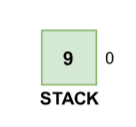• For the second iteration, i = 5, stack = , K = 3.
Since all the conditions for the while loop are true, i.e., K > 0, length of stack is greater than zero (1) and the top element of the stack is greater than i (9 > 5), we go inside the while loop.
(1) K becomes 2 as it is decremented by 1.
(2) The element 9 is removed from the stack.
(3) Finally, when we come out of the while loop, the digit 5 is added to the stack.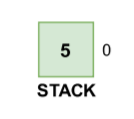• For the third iteration, i = 1, stack = , K = 2.
Since all the conditions for the while loop are true, i.e., K > 0, length of stack is greater than zero (1) and the top element of the stack is greater than i (5 > 1), we go inside the while loop.
(1) K becomes 1 as it is decremented by 1.
(2) The element 5 is removed from the stack.
(3) Finally, outside of the while loop, the digit 1 is added to the stack.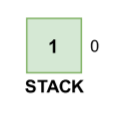• For the the fourth iteration, i = 6, stack = , K = 1.
Since one of the condition for the while loop is not true, i.e., the top element of the stack is not greater than i (1 !> 6), the while loop will be skipped and i (6) is directly added to the stack.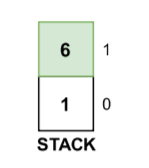• For the fifth iteration, i = 3, stack = [1, 6], K = 1.
Since all the conditions for the while loop are true, i.e., K > 0, length of stack is greater than zero (2) and the top element of the stack is greater than i (6 > 3), we go inside the while loop.
(1) K becomes 0 as it is decremented by 1.
(2) The element 6 is removed from the stack.
(3) Finally, when we come out of the while loop, the digit 3 is added to the stack.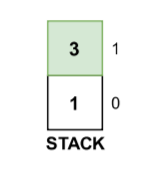• We have now completed the main loop of our algorithm and our stack = [1, 3].
If we combine these digits from the starting of the stack (0th index till nth index), we will get our final answer, i.e., 13. We can return this as our final answer.

• You might have noticed that we perform one more check after our main loop that checks if the value of K > 0.
We perform this check for corner cases for numbers such as 11111, 33333, etc. where there is no peak digit. If this condition is true, then we will simply remove K elements from the number and return it as our final answer.

# Time and Space Complexity

Time complexity:

We have a main loop in the algorithm that runs over n times, where n is the number of digits present in the input number. We also have another conditional loop inside the main loop that will run K times.

We know that each element is going to be processed at most twice with respect to the operations performed (1 push and 1 pop) and hence there will be 2n operations at most. This makes our complexity (2N).

Let us assume that a constant amount of work C is being done each time inside each loop (different operations such as comparison, insertion, removal, etc.), which makes our total complexity ((2N) + C).

We can ignore the constants.

Hence, the overall complexity becomes: `O(N)`.

Space complexity:

The length/size of the stack depends upon the input from the user, i.e., n.
Hence, the space complexity will be `O(N)`.

With this article at OpenGenus, you must have the complete idea of solving this problem of removing K digits to make smallest number using the idea of Monotonic Stack.#### Vansh Pratap Singh

Tough times don't last, tough people do. =)

Remove K digits to make smallest number